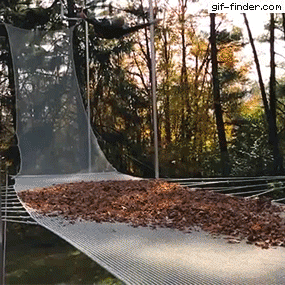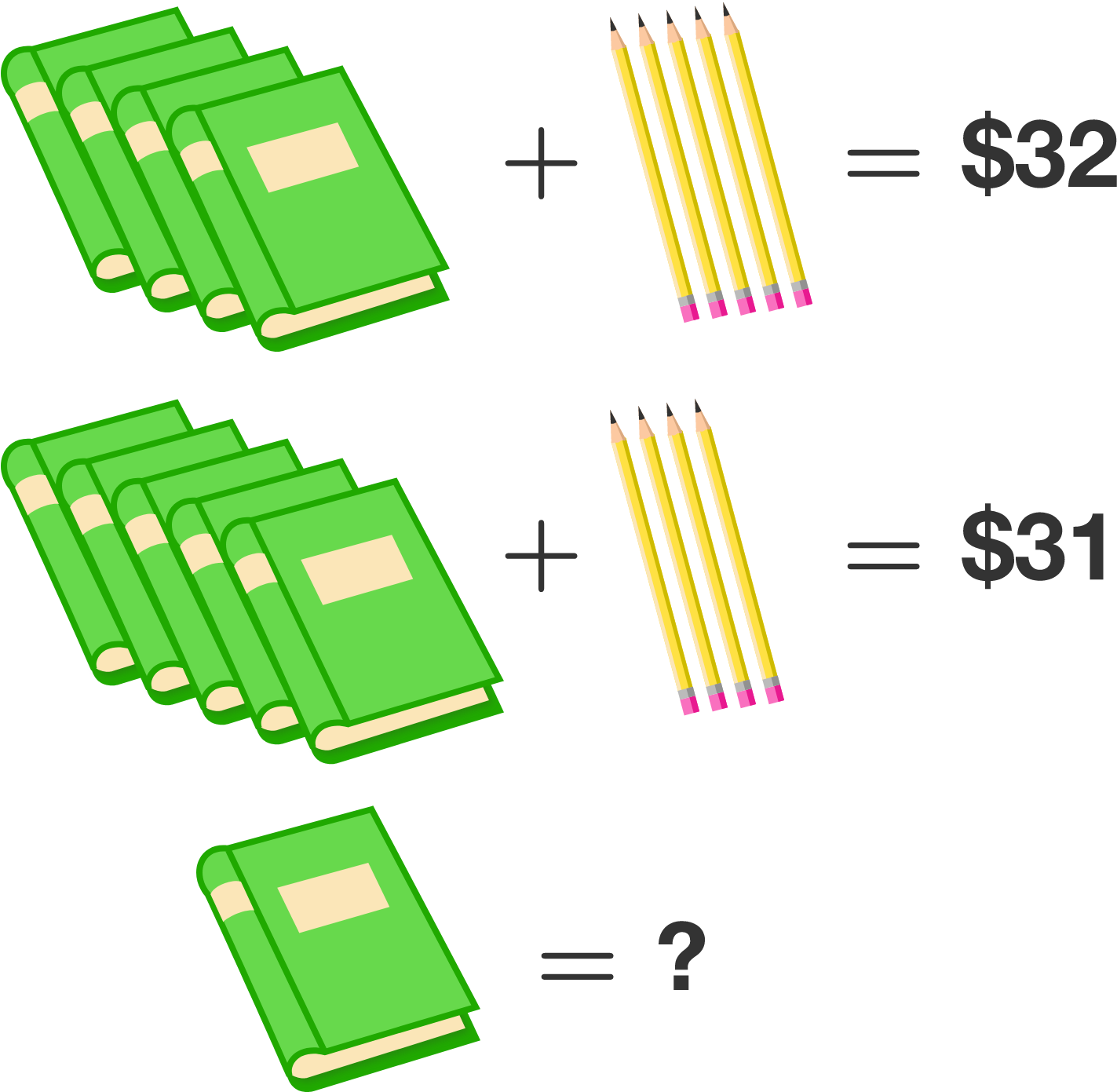# Problems of the Week

Contribute a problem

# 2017-10-02 BasicJim falls onto a net covered in leaves. The video reveals the mysterious motion of the leaves, which appear to stay in the air while the net below them stretches downwards.

Why don't the leaves fall along with the net?Some ice is floating in a glass and the temperature of the ice, water, and the surroundings are all equal to $0 \ ^\circ \text{C}.$

In which direction will heat flow between the ice and water?If the blue triangles continue to be formed indefinitely, what fraction of the big square will be shaded blue?In the polygon to the right, all adjacent edges are at right angles so that all its interior angles are either $90^\circ$ or $270^\circ.$

The four longer edges are equal in length, and all of the shorter edges are also equal in length. The area of the shape is 528.

What is the perimeter?

×